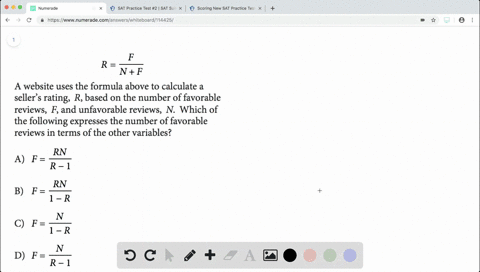Enroll in one of our FREE online STEM summer camps. Space is limited so join now!View Summer Courses### $$R=\frac{F}{N+F}$$ A website uses the formula a…

01:39Johns Hopkins University

Need more help? Fill out this quick form to get professional live tutoring.

Get live tutoring
Problem 11

$$7 x+3 y=8$$
$$6 x-3 y=5$$
For the solution $(x, y)$ to the system of equations
above, what is the value of $x-y ?$
$$\begin{array}{l}{\text { A) }-\frac{4}{3}} \\ {\text { B) } \frac{2}{3}} \\ {\text { C) } \frac{4}{3}} \\ {\text { D) } \frac{22}{3}}\end{array}$$

Check back soon!
SAT
SAT Practice Test # 6

## Discussion

You must be signed in to discuss.

## Video Transcript

contain. So for looking for the value of X minus pi, we first have to know the value of X and y. So if we look at these equations and I could notice that these are aligned and three y and night of three by would cancel out if we added these two equations. So I'm just gonna go ahead and do that if I added seven x close six x is thirty necks three wives last night of three wise and zero. So then I'm gonna leave three. Thirteen x on the left side and then eight plus five is also thirteen. So if I have thirteen axes you two thirteen and to by both sides by thirteen, clearly I got that X is equal to what? Okay, so I found that that's already. But once I know that, I just have to find my way back. So then I'LL have to do is take that one employment either one of the equations I'm going to go and choose to put put it into the first equation playing with your seven times one cause three y is equal to eight. So then I'm going to subtract seven from both sides to get three y is equal to one, and I'm going to divide those heads by three. Giving me that Y is equal to one third. So then, if I upload that one third right back into there, I get that one. Minus one third is the value that we're looking for, which was just two thirds B.